# CFO Estimation and Compensation¶

In the previous notebook, we have seen that a small carrier frequency offset (CFO) can have a large impact on the quality of the constellation diagram. Hence, we concluded that it's necessary to estimate and compensate any CFO that occurs during the transmission.

In this notebook, we will use the Schmidl&Cox metric to estimate and subsequently compensate the CFO with the aim of improving the constellation diagram in the audio channel. Before we start, let us quickly see mathematically how CFO influences a signal.

## Mathematical Foundations¶

Assume a baseband signal $x(t)$ with bandwith $B$ is upconverted using carrier frequency $f_c$. At the receiver side, it is downconverted using carrier frequency $f_c-\Delta_f$. Here, $\Delta_f$ describes the difference between the oscillators at the transmitter and receiver and hence mixing frequencies. Then, the downconverted signal $y(t)$ can be written as

$$y(t) = \exp\left(-j2\pi (f_c-\Delta_f)t\right)\exp\left(j2\pi f_c t\right) x(t)=\exp\left(j2\pi \Delta_f t\right)x(t)$$

Hence, the received signal is the transmitted signal multiplied by a complex exponetial. In the frequency domain we have

$$Y(f)=X(f-\Delta_f),$$

i.e. the received signal is a frequency-shifted version of the transmitted signal.

In their 1997 paper "Robust Frequency and Timing Synchronization for OFDM" Schmidl&Cox describe a technique for both time- and frequency offset estimation for OFDM. Before, we have already used their technique for the estimation of the start of the OFDM symbol in the time domain. In this notebook, we will use their metric to estimate the CFO. To recap, the metric $P(d)$ is given by

$$P(d)=\sum_{m=0}^{L-1} r[d+m]^*r[d+m+L].$$

Where $r[n]=x[n]\exp(j2\pi \Delta_f n/B)$ is the received signal and the transmitted signal $x[n]$ obeys the periodicity $x[n+L]=x[n]$ where $L$ is half of an OFDM symbol length. Hence we can write $r[d+m+L]=r[d+m]\exp(j2\pi \Delta_f \frac{T}{2})$, since $\frac{T}{2}=L/B$. So, the expression for $P(d)$ becomes

$$P(d)=\sum_{m=0}^{L-1} r[d+m]^*r[d+m]\exp\left(j\pi \Delta_f T\right)=\exp\left(j\pi \Delta_f T\right) \sum_{m=0}^{L-1}r[d+m]^*r[d+m]$$

Now, the sum above is a real number. Hence, the phase of $P(d)$ tells us something about the frequency offset:

$$\phi=\angle P(d)=\pi \Delta_f T$$

and hence we can estimate the frequency offset by

$$\hat{\Delta}_f=\frac{\phi}{\pi T}.$$

## CFO Estimation¶

Let us now gow ahead and check if above derivations numerically hold. First, some common imports:

In :
from audioComms.components import Component, TX, Environment, Recorder
from audioComms.plotting import PlotWaveform, PlotSpectrum, PlotConstellation, PlotBlock
from audioComms.channels import AudioChannel, SimulatedChannel
from audioComms.channels import IdentityChannelEffect, SimulatedChannelEffect, NoiseChannelEffect, InitialLossChannelEffect, ChainedChannelEffects, LoseSamplesChannelEffect


We import the PassbandChannel object. This object combines a real channel, the upconversion and the downconversion into one object to save some redundancy.

In :
from audioComms.passband import PassbandChannel


We also import specific OFDM blocks that have been developed in the previous notebooks:

In :
from audioComms.ofdm import OFDM, random_qam, ofdm_modulate, MostBasicOFDMReceiver, createSchmidlCoxPreamble
from audioComms.ofdm import BlockPilotRandomDataOFDMTransmitter, BlockPilotLSChannelEstimator, BlockPilotOFDMReceiver
from audioComms.synchronization import SchmidlCoxDetectPeaks, SchmidlCoxMetric


In a first attempt, we only record the P metric such that we can analyze it offline. BlockPilotRandomDataOFDMTransmitter(...) is the OFDM transmitter we have developed in the previous notebook. However, it's expanded to remove the pilot block from the transmision. Here, we dont need the pilots for now, as we are not going to estimate the channel yet. Instead, we only record the output of the S&C metric component.

In :
# Source code has been redacted in online version
# Omitting 17 lines of source code


Let's run this function with some CFO of e.g. 5Hz:

In :
B = 441*5*4  # baseband bandwidth
cfo = 5      # the experienced CFO
S = recordPMetric(SimulatedChannel, B=B, cfo=cfo)
M_metric = S
P_metric = S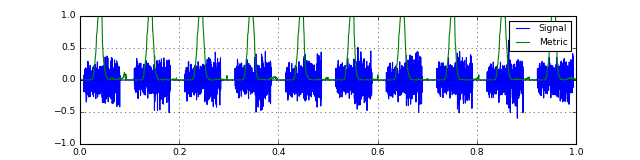Once we have recorded the metrics, let's see the overall metric and the angle of $P$.

In :
# Source code has been redacted in online version
# Omitting 4 lines of source code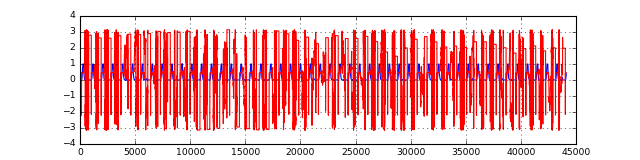Hm, there's nothing to be seen actually. Let's remember that in our previous derivation we assumed that $x[d]=x[d+L]$. However, this only holds during the transmission of the preamble, where the metric gets a high value. So, let us filter out any angle, where the metric is not high enough. Also, let's zoom a bit into the position where an actual metric peak occurs:

In :
plt.figure(figsize=(8,2))
useful_angle = np.angle(P_metric) * (abs(M_metric) > 0.9).astype(int)  # filter out parts where the metric is below 0.9

plt.subplot(121)
plt.plot(M_metric)
plt.plot(useful_angle, lw=2, color='r')
plt.grid(True); plt.ylim((-4,4))

plt.subplot(122)  # plot a zoomed version of above plot
plt.plot(M_metric)
plt.plot(useful_angle, lw=2, color='r')
plt.grid(True); plt.xlim((1000, 1400)); plt.ylim((-4, 4));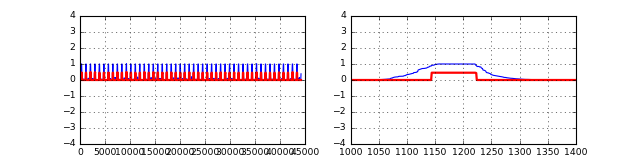Ah, now the signal is already a lot clearer. We can see that during the plateau of the peak, the phase of the P-metric remains constant. Let us now check, if we can infer the actual CFO from this metric using the formula $\hat{\Delta}_f=\frac{\phi}{\pi T}$:

In :
plt.figure(figsize=(8,2))
plt.plot(M_metric)
K = 256      # OFDM K (needs to match the value in recordPMetric(...))
T = K/B
plt.plot(useful_angle / (np.pi*T), color='r');
plt.grid(True); plt.ylim((-abs(cfo)-1, abs(cfo)+1));
plt.axhline(cfo, ls='--', color='k', lw=3); plt.xlabel('sample index'); plt.ylabel('Measured CFO');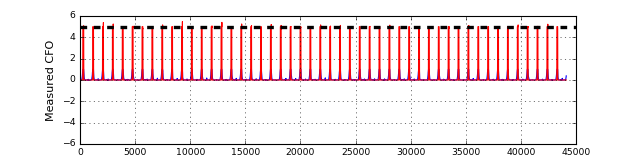Great! Using the Schmidl-Cox formula, we can clearly infer the frequency offset from the metric!

Let us now in the following write a component that measures the current CFO given the S&C P-metric:

In :
class CFOExtractor(Component):
"""This component expects to be called with the P-metric over the full OFDM frame. It then extracts the CFO phase
from the position of the plateau of the metric"""
def __init__(self, environment, ofdm, rate):
super().__init__(environment, None)
self._ofdm = ofdm
self._rate = rate

# The end of the plateau is at the end of the first OFDM symbol, i.e. at sample K+CP. Hence, we choose the center of the
# plateau for extraction of the CFO (i.e. K+CP/2 since the plateau consists of CP samples)
extractionPoint = self._ofdm.K + self._ofdm.CP // 2
c = P_metric[extractionPoint + np.arange(1)]

# convert the phase into an actual CFO value and forward it to the next components
self._send(np.angle(c)/(np.pi*self._ofdm.K)*self._rate, stream='CFO')


We can now include this component into an actual signal processing chain:

In :
def runCFOMeasurement(channelFunc, B=441*5*4, Fc=8000, cfo=0):

# Create the components
env = Environment(samplerate=44100)

# transmitter
tx = BlockPilotRandomDataOFDMTransmitter(env, ofdm, usePilotBlock=False)

# channel
chan = PassbandChannel(env, channelFunc=channelFunc, Fc=Fc, B=B, cfo=cfo)

# synchronization
scMetric = SchmidlCoxMetric(env, ofdm=ofdm, minEnergy=1)
scDetect = SchmidlCoxDetectPeaks(env, K=ofdm.K, CP=ofdm.CP, frameLen=frameLen)  # extract the frames based on the metric magnitude
cfoExtract = CFOExtractor(env, ofdm, B)

# visualization
fig = env.figure(figsize=(10,6))
showSpectrum = PlotSpectrum(env, windowDuration=0.3, logScale=1, axes=fig.add_subplot(211), xlim=(0, 44100/2), ylim=(-100, -40))
showMetric = PlotWaveform(env, axes=fig.add_subplot(223), signalTransform=abs, ylim=(-0.1, 2), title='Sync metric', numLines=2)
showCFOstream = PlotWaveform(env, axes=fig.add_subplot(224), integerXaxis=True, windowDuration=100, ylim=(-5,5), title='Measured CFO [Hz]')

# set up the connections
tx.transmitsTo(chan)
chan.transmitsTo(showSpectrum, stream='RF')
chan.transmitsTo(scMetric)

scMetric.transmitsTo(scDetect)
scMetric.transmitsTo(showMetric)

scDetect.transmitsTo(cfoExtract, stream='P_metric')

cfoExtract.transmitsTo(showCFOstream, stream='CFO')

env.run()


Let's run this chain, first in a simulated channel with a deterministic CFO of 2Hz:

In :
runCFOMeasurement(SimulatedChannel, cfo=2, B=441*5*4*1)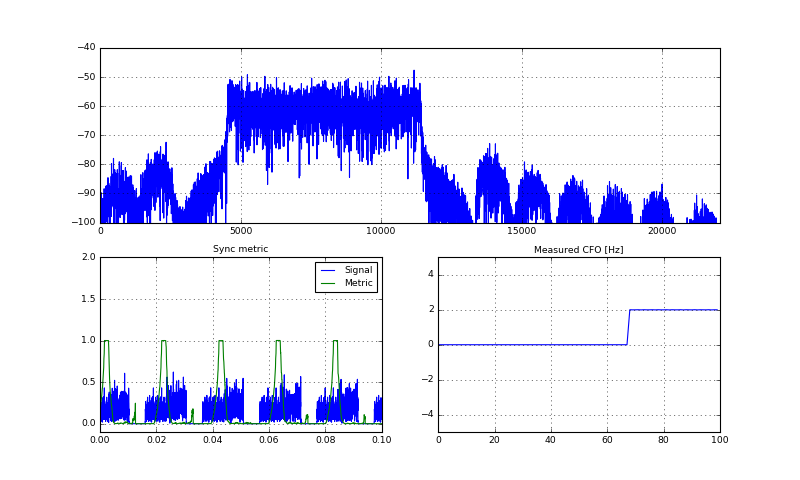Stop received from external


Yes, the CFO extractor works correctly, as it displays a 2Hz frequency offset in the plot. Now, let's look at the output of the chain when using the real audio channel. We on top add an artifical CFO of 2Hz again:

In :
runCFOMeasurement(AudioChannel, cfo=2, B=441*5*4)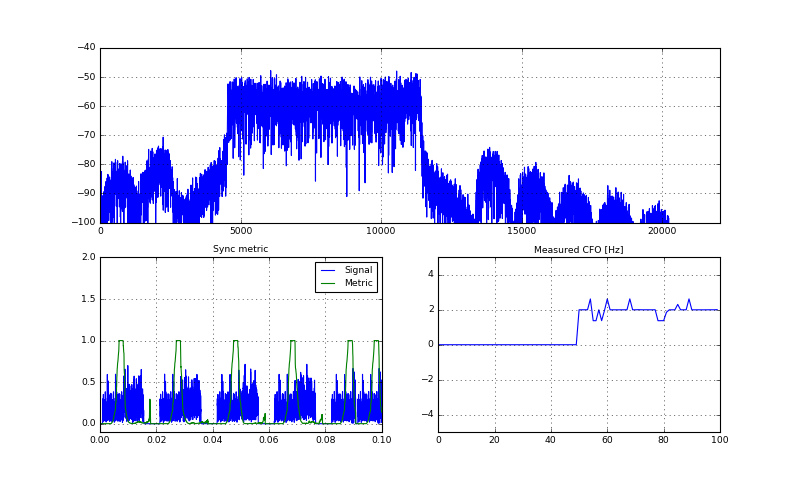Stop received from external


OK, after some initial jitter, the measured CFO also stabilizes at 2Hz. However, it does not seem that the audio channel itself introduces additionaly CFO. Though, when zooming into the CFO output, one can see a very small oscillation of the CFO over time.

## CFO Correction¶

Now, that we could correctly estimate the CFO, we are required to compensate or correct it before attempting demodulation. The CFO correction simpley applies the inverse operation

$$\hat{y}(t)=y(t)\exp\left(-j2\pi \hat{\Delta}_ft\right)=x(t)\exp\left(j2\pi (\Delta_f-\hat{\Delta}_f)t\right)$$

Hence, if we estimated correctly, the CFO is completely removed. Let's write an according component that takes one OFDM frame and corrects its CFO based on the last measurement:

In :
class CFOCorrection(Component):
def __init__(self, environment, rate, bypass=False):
super().__init__(environment, None)
self._rate = rate
self._bypass = bypass

self._currentCFO = 0

if stream == 'CFO':
# update with a new CFO measure
self._currentCFO = signal
elif stream == 'frame':
# correct a frame signal
if self._bypass:
self._send(signal, stream='frame')
else:
t = np.arange(len(signal))/self._rate
factor = np.exp(2j*np.pi*self._currentCFO*t)
self._send(signal*factor, stream='frame')
else:
raise RuntimeError("Unknown stream \"%s\"!" % stream)


We are now ready, to use our old OFDM chain and include the newly written CFO estimation and correction units:

In :
def runTransmissionWithCFOCorrection(channelFunc, B=441*5*4, Fc=8000, cfo=0, bypassCFO=False):

# Create the components
env = Environment(samplerate=44100)

# transmitter
tx = BlockPilotRandomDataOFDMTransmitter(env, ofdm, usePilotBlock=True)

# channel
chan = PassbandChannel(env, channelFunc=channelFunc, Fc=Fc, B=B, cfo=cfo)

# synchronization
scMetric = SchmidlCoxMetric(env, ofdm=ofdm, minEnergy=1)
scDetect = SchmidlCoxDetectPeaks(env, K=ofdm.K, CP=ofdm.CP, frameLen=frameLen)
cfoExtract = CFOExtractor(env, ofdm, rate=B)
cfoCorrect = CFOCorrection(env, rate=B, bypass=bypassCFO)

# Receiver, use the previously defined MostBasicChannelEstimation

# visualization
fig = env.figure(figsize=(10,6))
showSpectrum = PlotSpectrum(env, windowDuration=0.3, logScale=1, axes=fig.add_subplot(311), xlim=(0, 44100/2), ylim=(-100, -40))
showMetric = PlotWaveform(env, axes=fig.add_subplot(323), signalTransform=abs, ylim=(-0.1, 2), title='Sync metric', numLines=2)
showCFO = PlotWaveform(env, axes=fig.add_subplot(324), integerXaxis=True, windowDuration=100, ylim=(-5,5), title='Measured CFO')
showHest = PlotBlock(env, axes=fig.add_subplot(325), numLines=2, ylim=(-4,4), title='Channel Estimation')
showConstellation = PlotConstellation(env, axes=fig.add_subplot(326), title='Rx Constellation', numLines=5)

# set up the connections
tx.transmitsTo(chan)
chan.transmitsTo(showSpectrum, stream='RF')
chan.transmitsTo(scMetric)

scMetric.transmitsTo(scDetect)
scMetric.transmitsTo(showMetric)

scDetect.transmitsTo(cfoExtract, stream='P_metric')
scDetect.transmitsTo(cfoCorrect, stream='frame')

cfoExtract.transmitsTo(showCFO, stream='CFO')
cfoExtract.transmitsTo(cfoCorrect, stream='CFO')

cfoCorrect.transmitsTo(rx, stream='frame')
rx.transmitsTo(showHest, stream='Hest')
rx.transmitsTo(showConstellation, stream='constellation')

env.run()


Let's first run this with a CFO of 2Hz and see if the constellation is sharp:

In :
runTransmissionWithCFOCorrection(SimulatedChannel, cfo=2)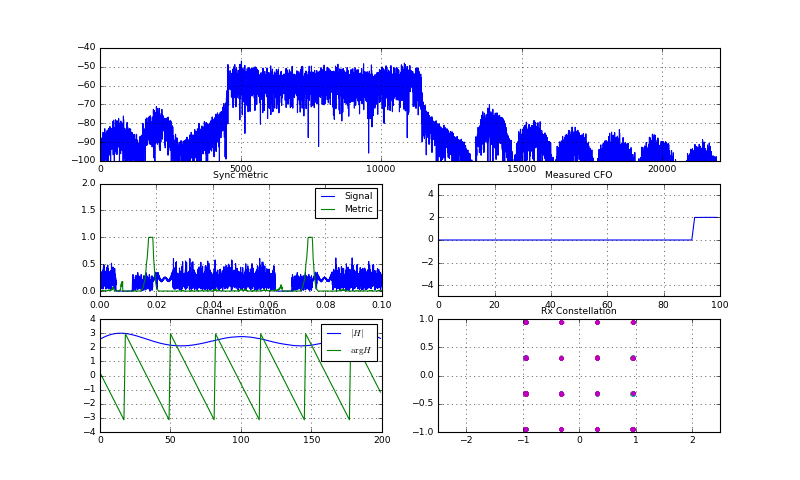Stop received from external


Yes, it seems that the CFO correction unit works. To verify, let's bypass the CFO correction and see the resulting constellation:

In :
runTransmissionWithCFOCorrection(SimulatedChannel, cfo=2, bypassCFO=True)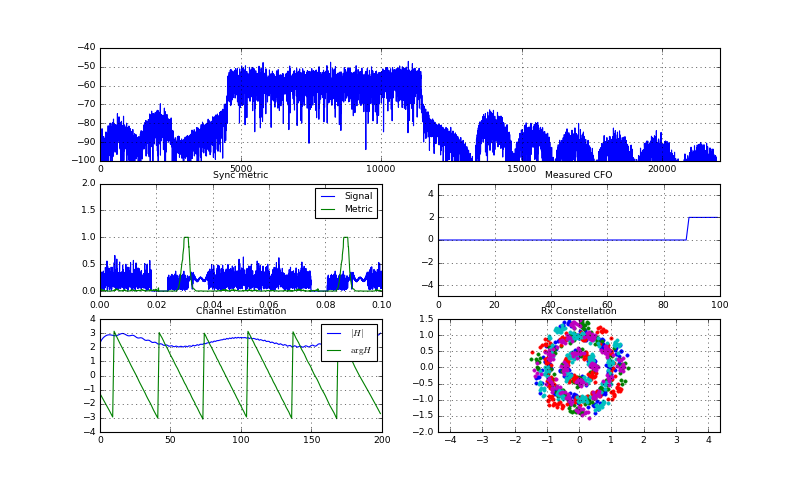Stop received from external


Yes, the constellation is now completely messed up due to CFO. Hence, we can conclude that in principle the CFO corrector works. We can now go ahead and see, if we could improve the constellation over the audio channel:

In :
runTransmissionWithCFOCorrection(AudioChannel, cfo=0)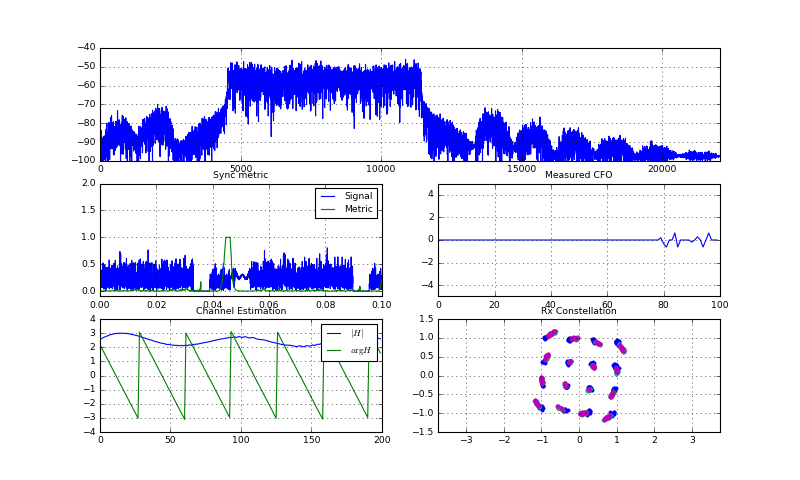Stop received from external


Well, not really: The constellation diagram has not significantly improved. Let us double-check by bypassing the CFO correction:

In :
runTransmissionWithCFOCorrection(AudioChannel, cfo=0, bypassCFO=True)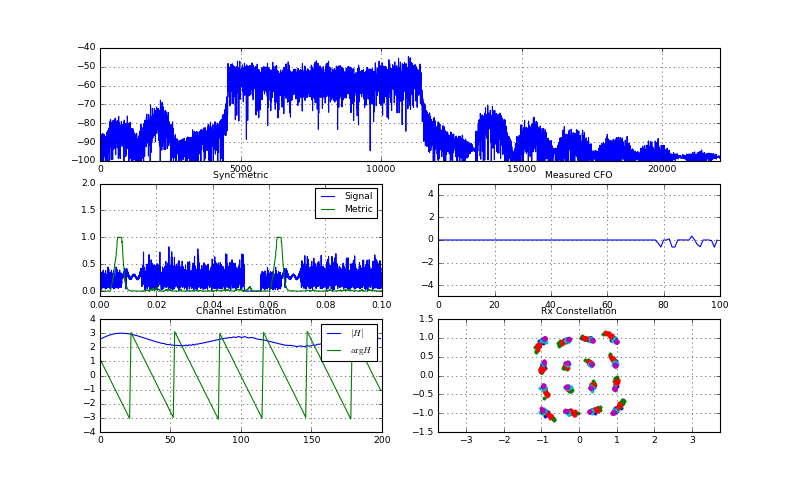Stop received from external
Stream Stopped 1
Stream Stopped 2


Hm, the constellation points have a similar behaviour with or without CFO correction. Hence, we need another method to improve the constellation diagram. Nevertheless, the correction of CFO is an important part in any OFDM system, as we cannot ensure that both transmitter and receiver exhibit no CFO. For example, transmitting from one soundcard to another might have a bit of CFO, when the sound cards are not synchronized enough.

## Conclusion¶

Unfortunately, the successful CFO correction did not really have the expected positive effect on the constellation diagram. Also, we could not measure a significant CFO when using the soundcard. So, the journey for a clearer constellation diagram goes on.

In the next notebook, we will again focus on channel estimation to improve the constellation diagram. We introduce a phase-correction pilot and comb-type pilots estimation procedure, which allows a better equalization in time-variant channels, as the audio channel is one.